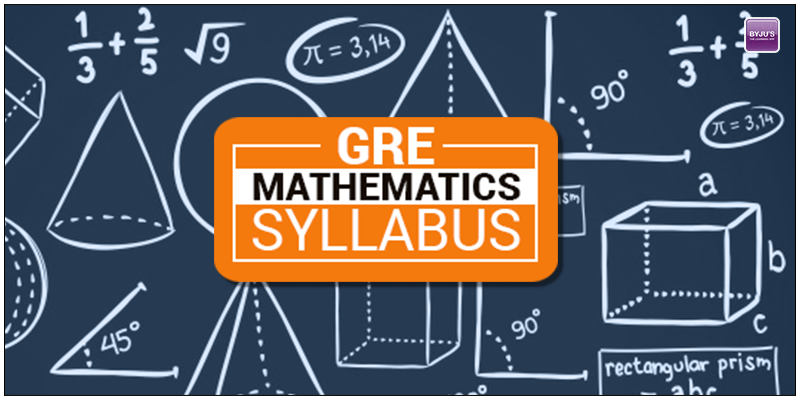# GRE Mathematics Syllabus for Subject TestOne of the major sections in the GRE test is the GRE Quantitative Reasoning. This majorly comprises of questions from Maths. The mathematics questions in the test are drawn from the undergraduate level courses. It consists of approximately 66 multiple-choice questions. About 50 percent of the questions comprises of calculus and its applications — subject matter that is assumed to be common to the backgrounds of almost all mathematics majors. Approximately 25 percent of the questions in the test are extracted from elementary algebra, linear algebra, abstract algebra and number theory.

Before appearing for the test, the aspirants should be well versed with the syllabus of the test. As it may be challenging for those who don’t belong to the mathematical background.

## Content Specifications

The following content descriptions may assist students in preparing for the test. The percentages given are estimates; the actual percentages will vary somewhat from one edition of the test to another.

#### ALGEBRA — 25%

• Elementary algebra: basic algebraic techniques and manipulations acquired in high school and used throughout mathematics
• Linear algebra: matrix algebra, systems of linear equations, vector spaces, linear transformations, characteristic polynomials, and eigenvalues and eigenvectors
• Abstract algebra and number theory: elementary topics from group theory, theory of rings and modules, field theory, and number theory

#### CALCULUS — 50%

Material learned in the usual sequence of elementary calculus courses — differential and integral calculus of one and of several variables — including calculus-based applications and connections with trigonometry,coordinate geometry, differential equations and other branches of mathematics.